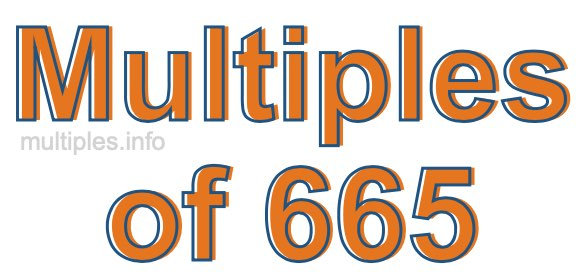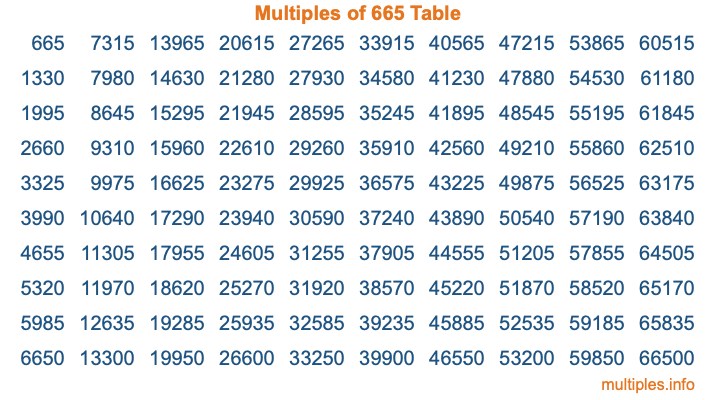Multiples of 665Welcome to the Multiples of 665 page. Here we will first teach you everything you will ever need to know about the multiples of 665, and then give you a study guide summary of everything we taught you to make sure you remember it all. Use this page to look up facts and learn information about the multiples of 665. This page will make you a multiples of six hundred sixty-five expert!

Definition of Multiples of 665
Multiples of 665 are all the numbers that when divided by 665 equal an integer. Each of the multiples of 665 are called a multiple. A multiple of 665 is created by multiplying 665 by an integer.

Therefore, to create a list of multiples of 665, you start with 1 multiplied by 665, then 2 multiplied by 665, then 3 multiplied by 665, and so on for as long as you want. Thus, the list of the first five multiples of 665 is 665, 1330, 1995, 2660, and 3325. To see a larger list of multiples of 665, see the printable image of Multiples of 665 further down on this page. We also have a category where you can choose any nth multiple of 665.

Multiples of 665 Checker
The Multiples of 665 Checker below checks to see if any number of your choice is a multiple of 665. In other words, it checks to see if there is any number (integer) that when multiplied by 665 will equal your number. To do that, we divide your number by 665. If the the quotient is an integer, then your number is a multiple of 665.

Is  a multiple of 665?

Least Common Multiple of 665 and ...
A Least Common Multiple (LCM) is the lowest multiple that two or more numbers have in common. This is also called the smallest common multiple or lowest common multiple and is useful to know when you are adding our subtracting fractions. Enter one or more numbers below (665 is already entered) to find the LCM.

Check out our LCM Calculator if you need more details about the Least Common Multiple or if you need the LCM for different numbers for adding and subtraction fractions.

nth Multiple of 665
As we stated above, 665 is the first multiple of 665, 1330 is the second multiple of 665, 1995 is the third multiple of 665, and so on. Enter a number below to find the nth multiple of 665.

th multiple of 665

Multiples of 665 vs Factors of 665
665 is a multiple of 665 and a factor of 665, but that is where the similarities end. All postive multiples of 665 are 665 or greater than 665. All positive factors of 665 are 665 or less than 665.

Below is the beginning list of multiples of 665 and the factors of 665 so you can compare:

Multiples of 665: 665, 1330, 1995, 2660, 3325, etc.

Factors of 665: 1, 5, 7, 19, 35, 95, 133, 665

As you can see, the multiples of 665 are all the numbers that you can divide by 665 to get a whole number. The factors of 665, on the other hand, are all the whole numbers that you can multiply by another whole number to get 665.

It's also interesting to note that if a number (x) is a factor of 665, then 665 will also be a multiple of that number (x).

Multiples of 665 vs Divisors of 665
The divisors of 665 are all the integers that 665 can be divided by evenly. Below is a list of the divisors of 665.

Divisors of 665: 1, 5, 7, 19, 35, 95, 133, 665

The interesting thing to note here is that if you take any multiple of 665 and divide it by a divisor of 665, you will see that the quotient is an integer.

Multiples of 665 Table
Below is an image of the first 100 multiples of 665 in a table. The table is in chronological order, column by column. The first column has the first ten multiples of 665, the second column has the next ten multiples of 665, and so on.The Multiples of 665 Table is also referred to as the 665 Times Table or Times Table of 665. You are welcome to print out our table for your studies.

Negative Multiples of 665
Although not often discussed or needed in math, it is worth mentioning that you can make a list of negative multiples of 665 by multiplying 665 by -1, then by -2, then by -3, and so on, to get the following list of negative multiples of 665:

-665, -1330, -1995, -2660, -3325, etc.

Multiples of 665 Summary
Below is a summary of important Multiples of 665 facts that we have discussed on this page. To retain the knowledge on this page, we recommend that you read through the summary and explain to yourself or a study partner why they hold true.

There are an infinite number of multiples of 665.

A multiple of 665 divided by 665 will equal a whole number.

665 divided by a factor of 665 equals a divisor of 665.

The nth multiple of 665 is n times 665.

The largest factor of 665 is equal to the first positive multiple of 665.

665 is a multiple of every factor of 665.

665 is a multiple of 665.

A multiple of 665 divided by a divisor of 665 equals an integer.

665 divided by a divisor of 665 equals a factor of 665.

Any integer times 665 will equal a multiple of 665.

Multiples of a Number
Here you can get the multiples of another number, all with the same attention to detail as we did for multiples of 665 on this page.

Multiples of
Multiples of 666
Did you find our page about multiples of six hundred sixty-five educational? Do you want more knowledge? Check out the multiples of the next number on our list!